# Practice Questions on EMF and Electric measurements for JEE Main and Advanced

Question 1
12 wires each of resistance RΩ are connected in the form of a skeleton cube as shown below in the figure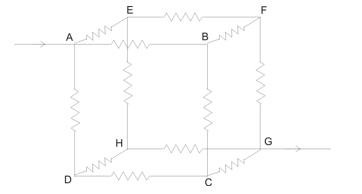If current I enter at point A and leaves at diagonally opposite point G then the equivalent resistance of the cube would be
1. 12R/5
2. 12R
3. 6R/5
4. 5R/6
Linked comprehension type question (A)
Consider the figure given below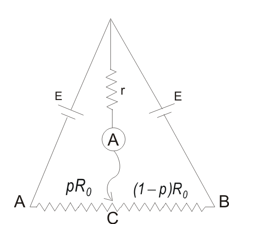Here AB is a wire of total resistance R0 . A jockey connected at point C can divide the wire into resistors of resistance pR0 and (p-1)R0 . Assuming that the batteries are identical and have zero internal resistance
Question 2
Current through ideal ammeter for any 0<p<1 is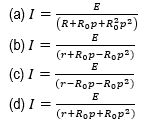Question 3
Value of p for which ammeter reads maximum value is
(a) 0
(b) 1
(c) 2
(d) 3

Linked comprehension type question (A)
Consider the circuit given below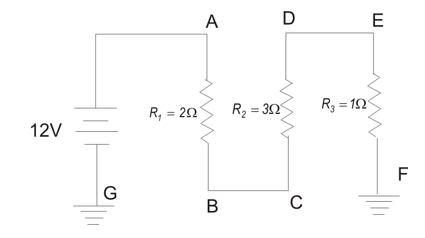Question 4
Total resistance in the circuit is
(a) 5Ω
(b) 6Ω
(c) (11/6)Ω
(d) 3Ω
Question 5
Current in the circuit is
(a) 4A
(b) 12A
(c) 6A
(d) 2A
Question 6
Potential difference between points A and E is
(a) 12V
(b) 6V
(c) 10V
(d) 5V
Question 7
Potential at point E is
(a) 10 V above the ground
(b) 10 V below the ground
(c) 2 V above the ground
(d) 2 V below the ground
Question 8
Power supplied by the battery is
(a) 12 W
(b) 10W
(c)22W
(d)24W

Matrix match type question
Question 9
Consider the two circuits given below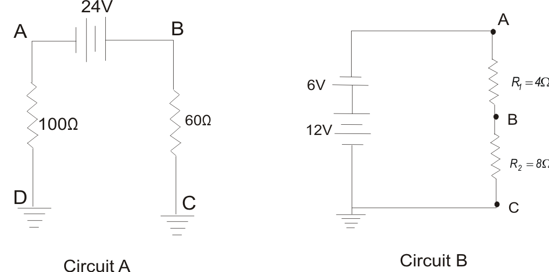Column A Column B (P) Point of lowest potential in circuit A has its value equal to (U) 0.15 A (Q) Circuit current in circuit B is (V) 4V (R) Circuit current in circuit A is (W) 15V (S) Potential of point B in circuit B is (X) 90V (T) Potential at point B in circuit A is (Y) .5A

Question 10
In the circuit shown below the reading of ammeter is the same when both switches open as both switches close.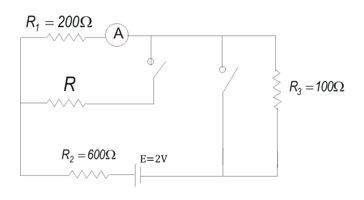The value of resistance R is
1. 800Ω
2. 900Ω
3. 1200Ω
4. 1000Ω
Linked comprehension type question (B)
For questions from 11 to 13 consider the statement given below
A 20µF capacitor which is initially uncharged is connected to a 6V battery through a resistor with resistance equal to 200Ω
Question 11
Magnitude of final charge q0 on the capacitor is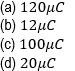Question 12
How long would it take for capacitor to be charged to (1/2)q0  after it is connected to the battery
(a) 3.8×10-3s
(b) 2.8×10-3s
(c) 2.8×10-4s
(d) 3.8×10-4s
Question 13
The time taken by the capacitor to be charged to 0.80q0 is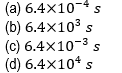Matrix match type question
Question 14
In the figure given below the circuit contains four capacitors having same capacitance of 10µF and a battery providing a voltage of 90V
Match the columns given below

 Column A Column B (P) When switch S2 is open and S1 is closed and then opened after C1 , C2 and C3 are fully charged. Electric potential difference across each capacitor is (W) 0 V (Q) Now after opening S1 if switch S2 is closed, the electric potential difference across each capacitor is (X) V1=V3=36V , V2=V4=18V (R) In another case if switch S1 is open and switch S2 is first closed then electric potential difference across each capacitor is (Y) V=30 V (S) Now if switch S1 is closed , the potential difference across each capacitor is (z) V1=V3=30V , V2=V4=15V

1. (d)
2. (b)
3. (a) , (b)
4. (b)
5. (d)
6. (c)
7. (c)
8. (d)
9. P—W ; Q—Y ; R—U ; S—V ; T—X
10. (c)
11. (a)
12. (b)
13. (c)
14. P—Y ; Q—Z ; R—W ; S—X

Solutions